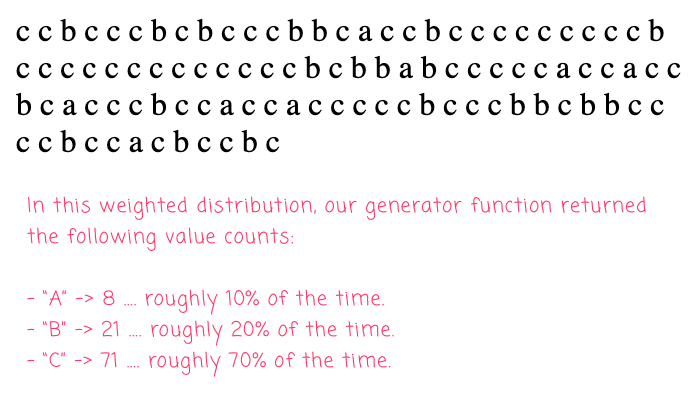# Using An Array To Power Weighted Distributions In Lucee CFML 5.3.8.201

By on
Tags:

Lately, I've been working on some code that needs to randomly assign a value to a request. But, the "randomness" isn't entirely random: the set values needs to be assigned using a weighted distribution. Meaning, over a period of time, each value should be "randomly assigned" a limited percentage of the time. I'm sure there are fancy / mathy ways to do this; but, I've found that pre-calculating an array of repeated values makes the value-selection process simple in Lucee CFML 5.3.8.201.

Imagine that I want to randomly select the following values with a weighted distribution:

• `A`: 10% of the time.
• `B`: 20% of the time.
• `C`: 70% of the time.

Instead of doing any fancy maths to figure this out, I'm literally taking those values and inserting them into a ColdFusion array, repeating each value according to the desired percent. So, if I want `A` to show up 10% of the time, I'm inserting `A` into the array 10-times. Then, I insert `B` 20-times and `C` 70-times. What this gives me is a ColdFusion array with 100 items and a composition that matches the weighted distribution.

At this point, in order to return a random weighted value, all I have to do is randomly select an index from this pre-calculated array. To see this in action, I'm going to code-up the above distribution and then select 100 random values:

``````<cfscript>

// Our build-function is going to return a generator function that produces values
// with the given weighted frequencies.
next = buildWeightedDistribution([
{ value: "a", percent: 10 },
{ value: "b", percent: 20 },
{ value: "c", percent: 70 }
]);

// By outputting 100 values, we should see occurrences that roughly match the defined
// percentages from above.
loop times = 100 {

echo( next() & " " );

}

// ------------------------------------------------------------------------------- //
// ------------------------------------------------------------------------------- //

/**
* I return a function that will produce values with the given distribution. Each entry
* is expected to have two properties:
*
* - value: the value returned by the generator.
* - percent: the weight (0-100) of the value in the distribution.
*/
public function function buildWeightedDistribution( required array distributions ) {

var index = [];
var indexSize = 0;

// In order to make it super easy to generate the next value in our series, we're
// going to pre-compute an array in which each value is repeated as many times as
// is required by its weight. So, a value that is supposed to be returned 30% of
// the time will be repeated 30 times in our internal index.
for ( var distribution in distributions ) {

loop times = distribution.percent {

index[ ++indexSize ] = distribution.value;

}

}

// Now that we have our internal index of repeated values, our generator function
// simply has to pick a random value from the index.
return(
() => {

return( index[ randRange( 1, indexSize, "SHA1PRNG" ) ] );

}
);

}

</cfscript>
``````

As you can see, I'm simply using the `CFLoop` tag, for each distribution, to repeat the given value the desired number of times. This leaves me with an array whose composition matches the weighted distribution. The returned fat-arrow function then does nothing more than select random values from the pre-calculated array. And, when we run this ColdFusion code, we get the following output:When this ColdFusion code ran, we ended up with the following value counts:

• `A`: 8 - which is roughly 10% of the time.
• `B`: 21 - which is roughly 20% of the time.
• `C`: 71 - which is roughly 70% of the time.

As you can see, our value counts roughly match the desired weighted distribution.

The main downside to this approach is that the values needed to be repeated in memory. But, memory is cheap, and array look-ups are fast. As such, this feels like a really nice solution to this approach in ColdFusion.

Want to use code from this post? Check out the license.Clever! I like it. And now I'm wondering how I would have handled it. Probably not as elegantly as you.

I'd love to know what the Mathy solution would look like out of curiosity, but as the saying goes...when all you have is a hammer, everything looks like a nail. And you (my friend) nailed it! 🤣@Chris,

😛 Honestly, I don't even know what the mathy solution would have been. The approach I outlined is the only way I know how to do it. I guess you could have called something like `rand()` to get a decimal between `0-1`; then, checked the decimal against the desired distributions ... but, that feels really complicated.@Ben

That was my first thought too, but then you'd have to track each range, which is additive...

A = 0 - 0.1
B = 0.11 - 0.3
C = 0.31 - 1

That does seem more complex. Even if your solution does more "work", in the end I love how easy it is to comprehend. It's just simple and straight forward.

But what if you're Amazon and you have product arrive at a distribution center that you need to ship out to various warehouses based on demand and you needed to do this more mathematically? You got me totally nerding out now! haha

I'm hoping there's some math nerd (meant in the most endearing way) out there following your blog though who will share some really cool formula with us. I'm excited!